Math resources Geometry Triangles

Equilateral triangle

# Equilateral triangle

Here you will learn about equilateral triangles, including what an equilateral triangle is and the properties of equilateral triangles.

Students first learn about triangles in kindergarten and 1st grade in geometry with their work in reason with shapes and their attributes. They expand their knowledge of triangles as they progress through elementary school and into secondary school.

## What is an equilateral triangle?

An equilateral triangle has three equal sides and three equal angles.

An equilateral triangle is the simplest of regular polygons because it has the fewest number of sides. Equilateral triangles can also be called equiangular triangles.

Properties of an equilateral triangle:

• All sides are equal length.
• All angles are equal in measure, 60^{\circ}.

The dashes on the sides and the angles of the triangle mean they are equal in measure or congruent.

An equilateral triangle is a special case of an acute triangle, because all the angles are acute and equal.

### Symmetry of equilateral triangles

An equilateral triangle has three lines of symmetry.

The lines go from one vertex to the middle of the opposite side.

Since equilateral triangles are also regular polygons, notice that the number of lines of symmetry equals the number of sides the polygon has.

### What is an equilateral triangle?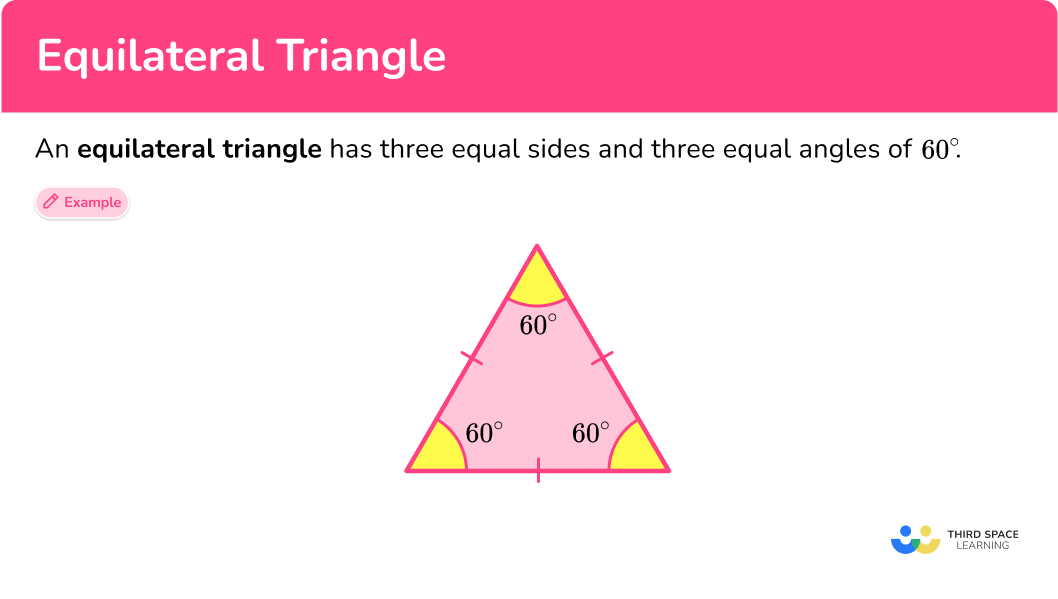## Common Core State Standards

• Grade 4 – Geometry (4.G.A.3)
Recognize a line of symmetry for a two-dimensional figure as a line across the figure such that the figure can be folded along the line into matching parts. Identify line-symmetric figures and draw lines of symmetry.

• Grade 5 – Geometry (5.G.B.3)
Understand that attributes belonging to a category of two-dimensional figures also belong to all subcategories of that category.

## How to identify an equilateral triangle

In order to classify triangles as equilateral triangles:

1. Recall the definition.
2. Explain why the triangle fits or does not fit the definition.

## Equilateral triangle examples

### Example 1: classify triangles

Is this triangle an equilateral triangle?

1. Recall the definition.

An equilateral triangle has three equal (congruent) sides and three (congruent) equal angles.

2Explain why the triangle fits or does not fit the definition.

This triangle has sides measuring 11 inches. Since the triangle has three equal sides all measuring 11 inches, it is an equilateral triangle.

### Example 2: classify triangles

Is triangle ABC an equilateral triangle?

Recall the definition.

Explain why the triangle fits or does not fit the definition.

### Example 3: classifying triangles

Are isosceles triangles also considered equilateral triangles?

Recall the definition.

Explain why the triangle fits or does not fit the definition.

### Example 4: classifying triangles

Are equilateral triangles also considered isosceles triangles?

Recall the definition.

Explain why the triangle fits or does not fit the definition.

### Example 5: classifying triangles

Every day, Teresa walks to school, and after school, she walks to her job at the local coffee shop and then walks home. Below is the path she takes. Does her path form an equilateral triangle?

Recall the definition.

Explain why the triangle fits or does not fit the definition.

### Example 6: classifying triangles

Skye drew the triangle below. What kind of triangle did Skye draw?

Recall the definition.

Explain why the triangle fits or does not fit the definition.

### Teaching tips for equilateral triangles

• Incorporate drawing activities where students can use rulers and protractors to create equilateral triangles.

• Incorporate origami activities that are creative and help students understand the properties of triangles, specifically equilateral triangles.

• Although worksheets have their place for practicing skills, using digital platforms for game playing is fun for students and helps them to practice skills. Check out free platforms such as desmos, quizlet, blooket, and quizziz.

### Easy mistakes to make

• Thinking that isosceles triangles can be classified as equilateral triangles
Isosceles triangles only have two equal (congruent) sides and angles. Equilateral triangles have three equal (congruent) sides and angles. So, equilateral triangles can be classified as isosceles triangles but isosceles triangles cannot be classified as equilateral triangles.

• Confusing angle facts
Make sure you know your angle facts.
• Acute angles are greater than 0^{\circ} and less than 90^{\circ}.
• Right angles equal 90^{\circ}.
• Obtuse angles are greater than 90^{\circ} and less than 180^{\circ}.

### Practice equilateral triangle questions

1. Which of these pictures shows an equilateral triangle?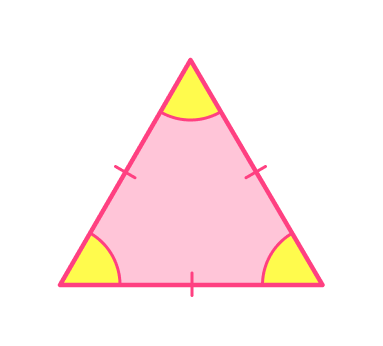The marks on the sides of the triangle show which lengths are equal.

This triangle was the only one that had all three angles and all three sides marked as being equal.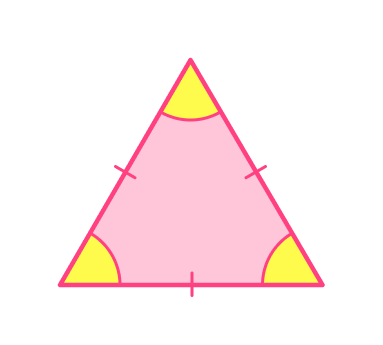2. How many lines of symmetry does an equilateral triangle have?

1236Equilateral triangles have three lines of symmetry.

An equilateral triangle is also a regular polygon, so the number of lines of symmetry is equal to the number of sides.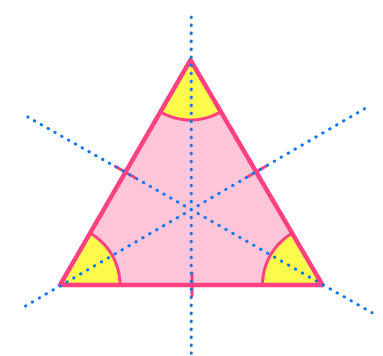3. Which of the following triangles is NOT an equilateral triangle?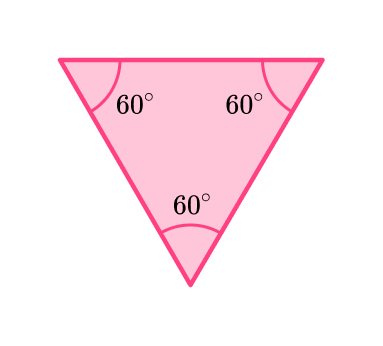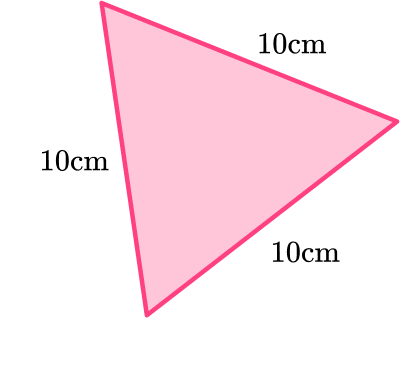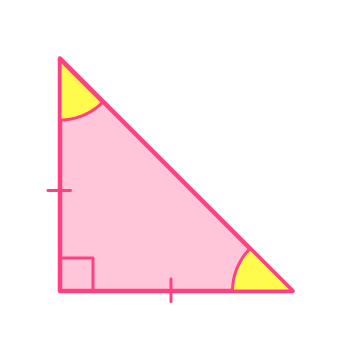An equilateral triangle has three equal sides and three equal angles.

This triangle has two equal sides and one 90^{\circ} angle.

So, it is not an equilateral triangle. It is an isosceles right triangle.4. Which picture below represents an equilateral triangle?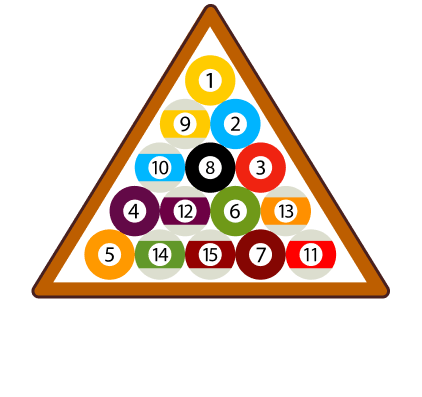The picture of the pool rack is the only image that appears to be an equilateral triangle.

It looks to have three equal (congruent) sides and angles.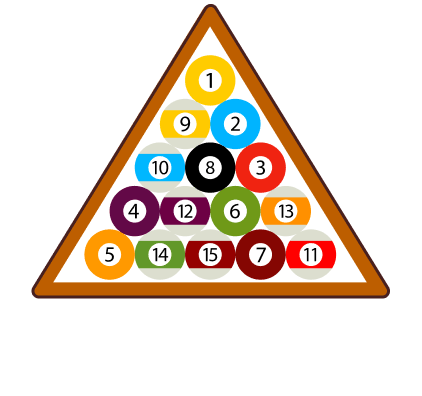5. The angle measures of a triangle are 60^{\circ}, 60^{\circ}, and 60^{\circ}. Which of the following best classifies the triangle?

Obtuse triangleIsosceles triangleEquilateral triangleRight isosceles triangleEquilateral triangles have three equal (congruent) sides and angles.

Each angle of an equilateral triangle is 60^{\circ}.

So, the best classification of a triangle with each angle being 60^{\circ} is an equilateral triangle.

6. An equilateral triangle will have ______ right angles.

0123Equilateral triangles have no right angles. Each angle of an equilateral triangle is equal in measure, which is 60^{\circ}.

## Equilateral triangle FAQs

Do equilateral triangles have equal exterior angles?

Yes, equilateral triangles have equal interior angles and equal exterior angles.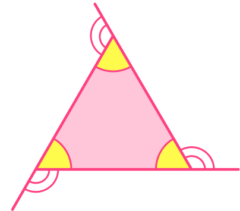Can you use the Pythagorean theorem on equilateral triangles to find a missing side length?

No, the Pythagorean theorem can only be used on right triangles to find missing side lengths.

Do equilateral triangles have a hypotenuse?

No, equilateral triangles do not have a side called the hypotenuse. The hypotenuse is the name of the longest side of a right triangle. So, only right triangles have hypotenuses.

How do you find the area of an equilateral triangle?

You find the area of an equilateral triangle using the formula for area of a triangle, A=\frac{1}{2} \cdot b \cdot h, where h, represents the height of an equilateral triangle.

How do you calculate the perimeter of an equilateral triangle?

You can add up the sides of an equilateral triangle to find the perimeter or take one of the side lengths and multiply it by 3 since they are all the same.

What are angle bisectors?

Angle bisectors are special line segments in a triangle that divide each angle in half. In middle and high school, you will learn about all the special segments of triangles (perpendicular bisectors, medians, angle bisectors, and altitudes).

Will triangles be studied in advanced math?

Properties of triangles will be further explored in high school to explore angles in parallel lines, circle theorems, trigonometry and Pythagoras’ theorem.

What is the altitude of an equilateral triangle?

The altitude of an equilateral triangle is the same thing as the height of an equilateral triangle.## Still stuck?

At Third Space Learning, we specialize in helping teachers and school leaders to provide personalized math support for more of their students through high-quality, online one-on-one math tutoring delivered by subject experts.

Each week, our tutors support thousands of students who are at risk of not meeting their grade-level expectations, and help accelerate their progress and boost their confidence.